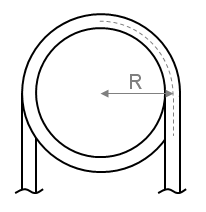# Summary

In order to determine the pressure drop in a pipe or coil the friction factor must first be calculated. This article presents the equations which may be used to determine the friction factor in coils and curved pipe.

# Definitions

 : Pipe Internal Diameter : Radius of coil helix (measured from axis to pipe centerline) : Dean number : Transitional Reynolds number for a coil : Friction factor for a coil of smooth pipe : Friction factor for rough pipe : Friction factor for smooth pipe

# Introduction

For single-phase flow in a coil or curved pipe a secondary flow pattern is established in the pipe which changes the fluid behavior and hence friction factor. Here we detail a method for estimating the Darcy friction factor for flow in coils for the purpose of calculating flow rate of pressure loss.

# Friction Factor Calculation

Note: All friction factors used here are Darcy/Moody friction factors (not fanning friction factors).

For single phase flow in a coil or curved pipe a secondary flow pattern is established in the pipe which changes the fluid behaviour and hence friction factor.

One effect is the stabilization of fluid flow, which results in an increase in the Reynolds number at which the flow enters the transition from laminar flow to turbulent flow. A Reynolds number correction is shown below, below this Re the fluid is in the laminar flow regime, above this Re the fluid is in the transitional or turbulent flow regime.

Here R is the radius of the coil helix, taken from the center of the pipe as shown below:To determine the friction factor in the coil the Dean number is required:

We can then calculate the friction factor for flow in a coil of smooth pipe.

For fully turbulent flow in smooth coils the correlation below may be used:

This equation is applicable in the ranges:

To account for the effect of roughness the following correction has been tentatively proposed:

For this correction we must know the friction factor for both rough and smooth straight pipe. We then determine the ratio of friction factors for smooth pipe and rough pipe at the diameter of the pipe making up the coil:

Using the ratio of friction factor for rough and smooth pipes we correct the friction factor determined previously for pipe made of smooth pipe. This is the friction factor to be used to calculate the flow rate or pressure drop for fluid flowing in coils or curved pipe.